Courses

# Control Unit (Advance Level) - 1

## 15 Questions MCQ Test Question Bank for GATE Computer Science Engineering | Control Unit (Advance Level) - 1

Description
This mock test of Control Unit (Advance Level) - 1 for Computer Science Engineering (CSE) helps you for every Computer Science Engineering (CSE) entrance exam. This contains 15 Multiple Choice Questions for Computer Science Engineering (CSE) Control Unit (Advance Level) - 1 (mcq) to study with solutions a complete question bank. The solved questions answers in this Control Unit (Advance Level) - 1 quiz give you a good mix of easy questions and tough questions. Computer Science Engineering (CSE) students definitely take this Control Unit (Advance Level) - 1 exercise for a better result in the exam. You can find other Control Unit (Advance Level) - 1 extra questions, long questions & short questions for Computer Science Engineering (CSE) on EduRev as well by searching above.
QUESTION: 1

### Which set of instruction transfers the memory word specified by the effective address to AC or Load to AC?

Solution:

LDA AC load to AC instruction transfers the memory word specified by the effective address to AC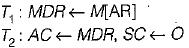Here register MD represent MDR.

QUESTION: 2

### Which of the following is not involved in a memory write operation?

Solution:

Program counter register used to read the value or instruction not in write operation. For write operation MAR, IR and MDR registers are used.

QUESTION: 3

### In Flynn’s classification of computers, the vector and array classes of machines belong to

Solution:
QUESTION: 4

Following are some statements associated with microprocessors. Identify the false statement.

Solution:

Instruction received by the CPU is decoded by control unit not by arithmetic unit.

QUESTION: 5

The following are four statements regarding what a CPU with only a set of 32 bit registers can perform.
1. Hold and operate on 32 bit integers.
2. Hold and operate on 16 bit integers.
3. Hold and operate on 64 bit floating point arithmetic.
4. Hold and operate on 16 bit UNICODE characters.

Which of the following is true about such a CPU?

Solution:

A CPU with only a set of 32 bit registers can perform:
1. Hold and operate on 32 bit integers.
2. Hold and operate on 16 bits integers.
3. Hold and operate on 16 bits Unicode characters.

QUESTION: 6

The following are four statements about Reduced Instruction Set Computer (RISC) architectures.
1. The typical RISC machine instruction set is small, and is usually a subject of a CISC instruction set.
2. No arithmetic or logical instruction can refer to the memory directly.
3. A comparatively large number of user registers are available.
4. Instructions can be easily decoded through hard-wired control units.

Which of the above statements is true?

Solution:

Reduced instruction set uses comparatively large number of user registers, all arithmetic or logical instruction refer to memory via registers only, instructions can be easily decoded through hardwired control unit and is small instruction set and is usually a subject of a CISI instruction set

QUESTION: 7

Consider an unpipelined processor assume that it has a 1 ns clock cycles and that it uses 4 cycles for ALU operation and branches and 5 cycles for memory operations. Assume that the relative frequencies of these operations are 40%, 20% and 40% respectively. Suppose due to clock skew and setup, pipelining the processor adds 0.2 ns of overhead to the clock, ignore any latency impact.

Q. What is the average instruction execution time for unpipelined processor?

Solution:

Average instruction execution time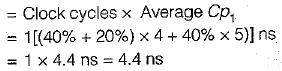QUESTION: 8

Consider an unpipelined processor assume that it has a 1 ns clock cycles and that it uses 4 cycles for ALU operation and branches and 5 cycles for memory operations. Assume that the relative frequencies of these operations are 40%, 20% and 40% respectively. Suppose due to clock skew and setup, pipelining the processor adds 0.2 ns of overhead to the clock, ignore any latency impact.

Q. What speedup gain after pipelined the processor?

Solution:

Average instruction time pipelined = 1 + 0.2 = 1.2 ns
Speedup from pipelined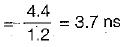QUESTION: 9

The following are the some of the sequences of operations in instruction cycle, which one is correct sequence?

Solution:

1. PC → MAR
2. M[MAR] → MDR
PC + 1 → PC
3. M[MDR] → IR
Represents the instruction fetch cycle

QUESTION: 10

Consider the following situation and fill in the blanks:

The computer starts the tape moving by issuing a command: the processor then monitors the status of the tape by means of a _____. When the tape is in the correct position, the processor issues a _______.

Solution:

Control command: A control command is issued to activate the peripheral and to inform it what to do.
Status: A status command is used to test various status conditions in the interface and the peripheral.
Data output command causes the interface to respond by transferring data from the bus into one of its register.

QUESTION: 11

Asynchronous data transfer between two independent units requires that control signals be transmitted between the communicating units to indicate the time at which data is being transm itted, way of achieving this ______.

Solution:

A synchronous data transfer between two independent units requires that control signals be transmitted between the communicating units to indicate the time at which data is being transmitted.
Two way of achieving this:
1. By means of a strobe pulse supplied by one of the units to indicate to the other unit when the transfer has to occur.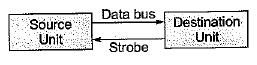2. To accompany each data item being transferred with a control signal that indicates the presence of data in the bus. The unit receiving the data item responds with another control signal to acknowledge receipt of the data. This type of agreement between two independent units is referred to as handshaking.

QUESTION: 12

Determine the width of Micro-instruction having following Control signal field, in a Vertical Microprogrammed Control Unit
1. Next Address field of 7 Bits
2. ALU Function field selecting 1 out of 13 ALU Function.
3. Register-in field selecting 1 out of 13 ALU Function.
4. Register-out field selecting 1 out of 8 registers.
5. Shifter field selecting no shift, right shift or left shift.
6. Auxiliary control field of 4 bits.

Solution:

Next Address Field = 7 Bits

ALU Function Field is of 4 Bits (to select possible 1 of 13 ALU fns)
Register-in and Register-out Fields of 3 Bits each Shifter Field of 2 bits to select 1 out of three possibilities:
Auxiliary Field of 4 Bits
Total Bits in μ-instruction
= 7 + 4 + 3 + 3 + 2 + 4 = 23

QUESTION: 13

Which statement is false in case of microprogram control?

Solution:
QUESTION: 14

The disadvantage of hard-wired control units with flip-flops is ______.

Solution:

The disadvantage of hardwired control units with flip-flops is that it requires more number of flip- flops.

QUESTION: 15

A CPU has a cache with block size 64 bytes. The main memory has k blocks, each block being c bytes wide. Consecutive c-byte chunks are mapped on consecutive blocks with warp-around. All the k blocks can be accessed in parallel, but two accesses to the same block must be serialized. A cache block access may involve multiple iterations of parallel block accesses depending on the amount of data obtained by accessing all the k-blocks in parallel. Each iteration requires decoding the block numbers to be accessed in parallel and this takes k/2 ns.
The latency of one block access is 80 ns. If c = 2 and k = 24, then latency of retrieving a cache block starting at address zero from main memory is

Solution:

Cache block size = 64 bytes
Main memory has K banks or k = 24
Each bank is 2 byte long because c = 2
Total time for one parallel access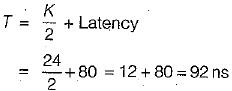Total latency time = CT
= 2 x 92 = 184 ns